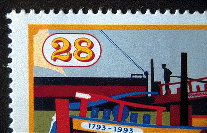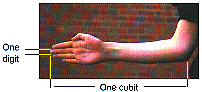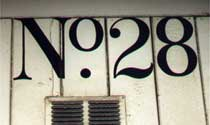# 28

= 2 × 2 × 7

= 2 × 14
= 4 × 7

= 1 + 2 + 3 + 4 + 5 + 6 + 7
A triangular number.

There are 28 days in February, except in leap years.

28 is a perfect number because it equals the sum of all its factors (apart from 28 itself).There are 28 digits in one cubit. The ancient Egyptians had their own system for measuring length based on body lengths. A digit was based on the width of one finger (about 19 mm). A cubit was the distance measured from the elbow to the tips of the fingers, and this equalled 28 digits.

Use a ruler to measure a digit and a cubit on yourself. How many of your digits make one cubit? How close is it to 28? Take measurements from a number of different people. How close are they to 28?